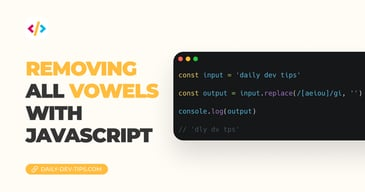Subscribe

# Vanilla JavaScript Comparison Operators

✍️

Lets dive into all the possible operators in JavaScript

9 Jun, 2020 · 2 min read

Yesterday we looked at the difference between the == and === comparison operator. These are used to compare two values or objects. But there are more comparison operators in `JavaScript` we can leverage.

Within `JavaScript`, we can leverage the following comparison operators. I've written down a table below to have a quick reference.

OperatorDescriptionComparingReturn
`==`Equal to`x == 10`
`x == 3`
`x == "3"`
false
true
true
`===`Equal value and type`x === 3`
`x === "3"`
true
false
`!=`Not equal`x != 10`
`x != "3"`
true
false
`!==`Not equal value and type`x !== "3"`
`x !== 3`
true
false
`>`Bigger than`x > 2`true
`<`Smaller than`x < 4`true
`>=`Bigger than or equal`x >= 3`true
`<=`Smaller than or equal`x <= 3`true

The == and === we discussed in detail yesterday.

### JavaScript != and !== Comparison permalink

As you can guess, these are very similar to the == and === but the ! Means `not` in most programming languages. So these will compare if the value is NOT what we compare it to. And the !== will even check for the correct type.

``````x = 3;

<!-- != comparison -->
console.log(x != 10) // true
console.log(x != "3") // false

<!-- !== comparison -->
console.log(x !== "3") // true
console.log(x !== 3) // false``````

As you can see in the second example it will think the string number three is wrong.

### JavaScript Smaller and Bigger Than Comparison permalink

The next ones are all in regards to smaller than or bigger than:

``````<!-- > comparison -->
console.log(x > 2) // true

<!-- < comparison -->
console.log(x < 4) // true

<!-- >= comparison -->
console.log(x >= 3) // true

<!-- <= comparison -->
console.log(x <= 3) // true``````

So note `>` is just for bigger than and `>=` also includes the actual number itself. Same goes for `<` and `<=`.

Feel free to explore the following Codepen.

See the Pen Vanilla JavaScript Comparison Operators by Chris Bongers (@rebelchris) on CodePen.

Tweet this tip

### Removing all vowels with JavaScript

3 Dec, 2022 · 2 min read### 10 games to learn JavaScript

2 Dec, 2022 · 3 min readJoin 2088 devs and subscribe to my newsletter

• 1000 articles written
• 2088 devs subscribed
• 529116 words written